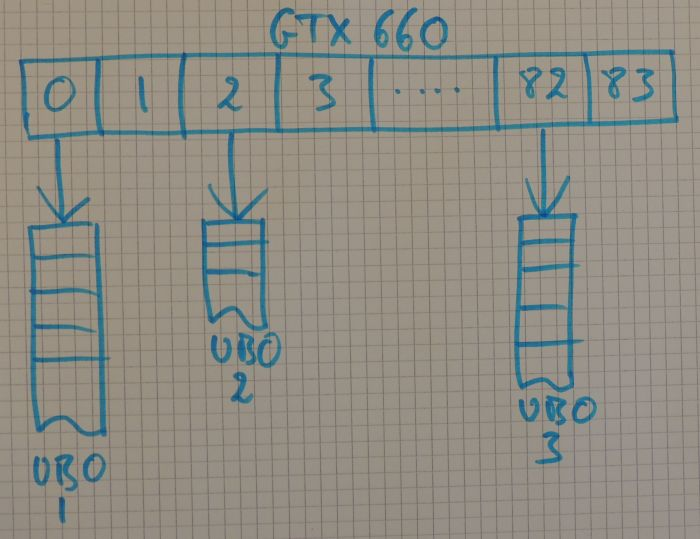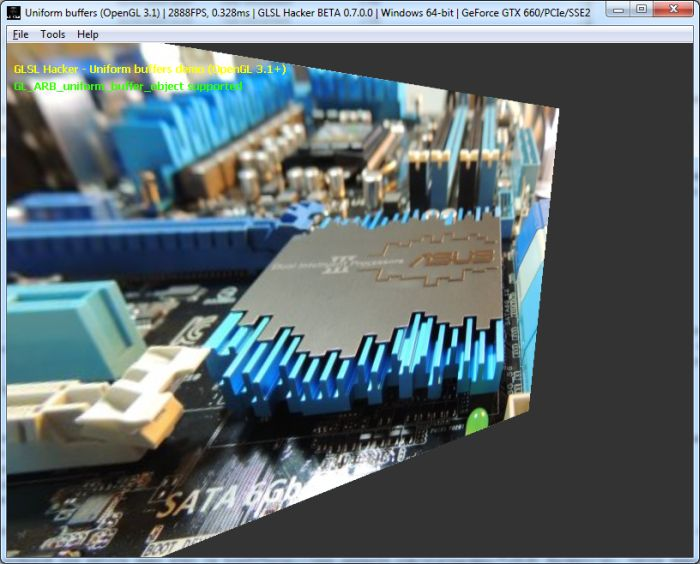# GPU Buffers: Introduction to OpenGL 3.1 Uniform Buffers Objects## 1 – Introduction

 Uniform Buffer Objects (or UBO in short) have been introduced with OpenGL 3.1. The uniform buffers bible can be found here: GL_ARB_uniform_buffer_object. Uniform buffers are memory zones allocated in the video memory of the graphics card (they are GPU buffers) and allow to pass data from host application to GLSL programs. The main advantage of using uniform buffers is that they can be shared between several GLSL shaders. Then, a single UBO is enough for all shaders that use the same data. From a GLSL shader point of view, an uniform buffer is a read-only memory buffer.

Let’s take a simple example: we need to pass to a shader the position of the camera, the position of a light as well as the light diffuse color. Using regukar uniform variables, we have the following inputs:

```uniform vec4 camera_position;
uniform vec4 light_position;
uniform vec4 light_diffuse;
```

For all shaders that need these variables, we have to update all uniform variables of each and every shader (with calls to glUniform4f() in OpenGL or gh_gpu_program.uniform4f() with GLSL Hacker. If there is only one shader, there’s no particular problem, but if there are 10 or even 100 different shaders, it’s more annoying. For variables that require frequent updates like the camera position (or transformation matrices), there must be a better way to update them once.

An uniform buffer is a simple and efficient solution. In the host application (C/C++), we use the following data structure:

```struct shader_data_t
{
float camera_position;
float light_position;
float light_diffuse;
```

Then we create an UBO from this data structure. Once the UBO is created, initialized with data and bound (like for any other kind of OpenGL buffer object), we can read data from this GPU buffer inside a shader using the following declaration:

```#version 150
...
{
vec4 camera_position;
vec4 light_position;
vec4 light_diffuse;
};
...
void main()
{
...
}
```

In this code snippet, shader_data is an uniform block (or more generally: an interface block as we’lll see it in an upcoming article).

We just need to update once per frame (if needed) this UBO and all shaders that read this buffer will have up to date uniform variables.

Each OpenGL rendering context can have only a limited number of UBOs bound at the same time. With a GeForce GTX 660 for example, we can bound at the same time up to 84 UBOs. Each GLSL shader (vertex, pixel, compute, tessellation or geometry) can have up to 14 uniform blocks for a GTX 660. The sum of all shader uniform blocks for a GTX 660 is 84…

An UBO has a limited size as well. With the GTX 660, the size of an UBO can not exceed 64KB or 65536 bytes.

These values (84, 14, 65536) are GPU-dependent limits. We can query OpenGL about these limits with the glGetIntegerv() function:

```GeForce GTX 660 limits (R337.50):
- GL_MAX_UNIFORM_BUFFER_BINDINGS -> 84
- GL_MAX_UNIFORM_BLOCK_SIZE -> 65536
- GL_MAX_VERTEX_UNIFORM_BLOCKS -> 14
- GL_MAX_FRAGMENT_UNIFORM_BLOCKS -> 14
- GL_MAX_GEOMETRY_UNIFORM_BLOCKS -> 14
```

For an Intel HD Graphics 4600, we have:

```Intel HD Graphics 4600 limits (v3652):
- GL_MAX_UNIFORM_BUFFER_BINDINGS -> 84
- GL_MAX_UNIFORM_BLOCK_SIZE -> 16384
- GL_MAX_VERTEX_UNIFORM_BLOCKS -> 14
- GL_MAX_FRAGMENT_UNIFORM_BLOCKS -> 14
- GL_MAX_GEOMETRY_UNIFORM_BLOCKS -> 14
```

And for an AMD Radeon HD 7970:

```AMD Radeon HD 7970 limits (Catalyst 14.4):
- GL_MAX_UNIFORM_BUFFER_BINDINGS -> 75
- GL_MAX_UNIFORM_BLOCK_SIZE -> 65536
- GL_MAX_VERTEX_UNIFORM_BLOCKS -> 15
- GL_MAX_FRAGMENT_UNIFORM_BLOCKS -> 15
- GL_MAX_GEOMETRY_UNIFORM_BLOCKS -> 15
```

## 2 – OpenGL Details

Let’s see how to create and update an uniform buffer.

Creation and initialization:

```GLuint ubo = 0;
glGenBuffers(1, &ubo);
glBindBuffer(GL_UNIFORM_BUFFER, ubo);
glBindBuffer(GL_UNIFORM_BUFFER, 0);
```

UBO update: we retrieve a pointer on the GPU memory (this operation is called the mapping) and we simply do a memory to memory copy:

```glBindBuffer(GL_UNIFORM_BUFFER, gbo);
GLvoid* p = glMapBuffer(GL_UNIFORM_BUFFER, GL_WRITE_ONLY);
glUnmapBuffer(GL_UNIFORM_BUFFER);
```

Now, let’s see how to make connections between an UBO and a GLSL program. The first step is to find the index of the uniform block in the shader:

```unsigned int block_index = glGetUniformBlockIndex(program, "shader_data");
```

The second step is to connect the uniform block to the UBO. We connect the interface block with the UBO using the uniform buffer binding point index. By default, if you only call glBindBuffer(GL_UNIFORM_BUFFER, gbo), the UBO is bound to the first binding point (index = 0). If we need it, and it’s mandatory if we have more than one UBO, we can specify a particular binding point for an UBO.

The specification of an binding point (here index = 2) is done with:

```GLuint binding_point_index = 2;
glBindBufferBase(GL_UNIFORM_BUFFER, binding_point_index, ubo);
```

We could have bound the UBO on binding point 23 or even 80. The max number of binding points can be found via GL_MAX_UNIFORM_BUFFER_BINDINGS. For the GeForce GTX 660, there are 84 possible binding points in the same OpenGL context. OpenGL holds a kind of array of pointers on the different uniform buffers. Each entry of this array is a binding point. For the GTX 660, this array has 84 elements. The following picture shows the uniform buffer binding point table of a GTX 600 with three UBOs bound on point 0, 2 and 82:Once the UBO is bound on a binding point, we can do the connection between the UBO and the shader:

```GLuint binding_point_index = 2;
glUniformBlockBinding(program, block_index, binding_point_index);
```

I think I covered the basic notions about uniform buffer objects. We know what is an UBO and how to manage it and read its content from a GLSL shader.

## 3 – Demo and References

I coded a little demo with GLSL Hacker that draws a textured quad with a GLSL program. Nothing special. Things get interesting if we look at how camera matrices are sent to the shader: with an uniform buffer. This demo is available in the host_api/gl-310-arb-uniform-buffer/ folder of GLSL Hacker demopack.

In GLSL Hacker 0.7.0+, I added a new Lua/Python library called gh_gpu_buffer. This low level lib allows to manages all kind of GPU buffers including uniform ones.Original article (french)
Buffers GPU: Les Uniform Buffers Objects d’OpenGL 3.1

External references
Shared Uniforms
GLSL Core Tutorial – Uniform Blocks

## 4 thoughts on “GPU Buffers: Introduction to OpenGL 3.1 Uniform Buffers Objects”

1.John Smith

GL_MAX_UNIFORM_BLOCK_SIZE = 65535 on my Radeon R9 280X with Catalyst 14.6 beta. R9 280x is the same as 7970

2.JeGX Post Author

Same thing here with GLSL Hacker + Cat 14.4, don’t know where I took the 16KB for the HD 7970. Probably a dirty cpy/pst from the Intel data. Article updated!

3.Jezeus

Have you benched Uniform blocks VS traditional unforms ? Our internal benches surprisingly did not show a real difference. Hopefully this is due to the youngness of the driver… because this concept was lacking since a while but if there is no performance gain, it unfortunately becomes useless. I will be happy to see a bench showing me that I am wrong.

4.casper

That purely depends on usage scheme. The main point of uniform buffers is that you don’t have to set uniforms per draw call, but only per frame. And if you have alot of them and you are CPU bound, then performance gain will be noticable in CPU part.